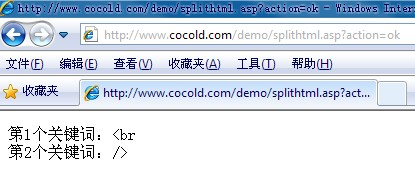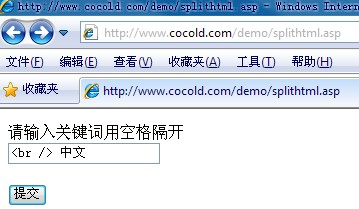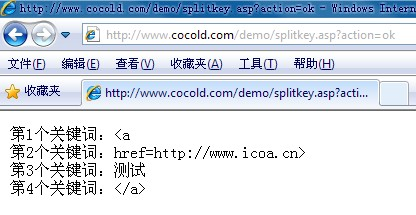# 将html代码转换为文本格式输出

```<% ok=request.QueryString("action") txt=server.htmlencode(Request.Form("txt")) if ok="ok" then %> <% str=Split(txt," ",-1) %> <%for i=0 to ubound(str)%> 第<%=(i+1)%>个关键词：<%=str(i)%><br> <%next%> <%else%> <form action="splitkey.asp?action=ok" method="post"> <p>请输入关键词用空格隔开<br /> <input type="text" name="txt" /></p> <input type="submit" value="提交" /> </form>```

``` ```

`<%end if%>````<% function HTMLEncode(fString) fString=replace(fString,"<","&lt;") fString=replace(fString,">","&gt;") HTMLEncode=fString end function %>```